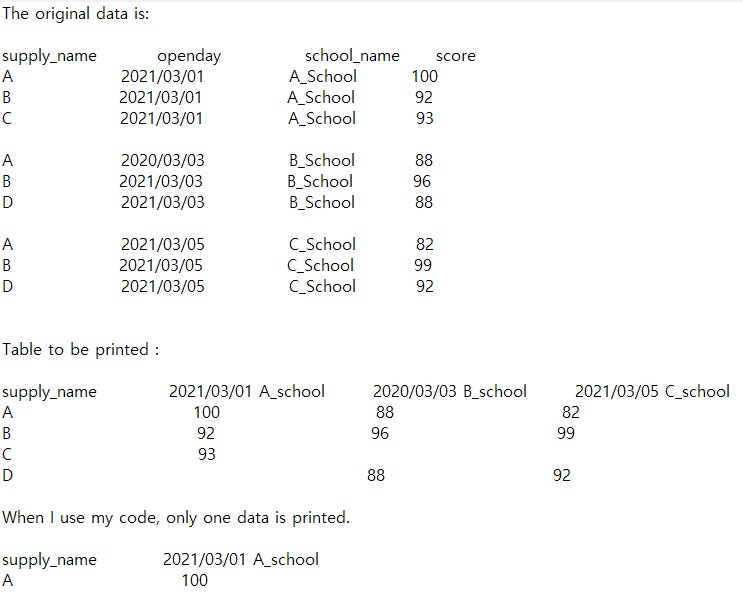# convert column to row. is it possible?

I want to make 2 columns(openday, school_name) into 1 row and print it as a table.I would like to print it out like a desired table.

Is it possible?

If possible, please explain in detail.

my code is :

``````<?php
\$SQL= "SELECT Concat_ws('\n' openday,'', school_name) AS school_column, supply_name,score  from result ";

\$grouped = [];
\$columns = [];

\$resultObject = \$result = mysqli_query(\$conn, \$SQL);
foreach (\$resultObject as \$row) {
\$grouped[\$row['supply_name']][\$row['school_column']] = \$row['score'];
\$columns[\$row['school_column']] = \$row['school_column'];
}

sort(\$columns);
\$defaults = array_fill_keys(\$columns,'-');
array_unshift(\$columns, 'school_column');

?>

<body>

<table id="example" class="display" style="width:100%" align="center">

<?php

While(\$result = mysqli_fetch_array(\$resultObject));
{
echo "<tr>
<td>supply_name</td>
<td> ".\$row['school_column']." </td>
</tr>";
}
?>

<tbody>

<?php

While(\$result = mysqli_fetch_array(\$resultObject));
{
echo "<tr>
<td> ".\$row['supply_name']." </td>
<td> ".\$row['score']." </td>

</tr>";
}

?>
</tbody>
</table>
</body>
``````